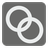Break Rings
Challenging
English ES FR JA RUA blacksmith gave his apprentice a task, ordering them to make a selection of rings. The apprentice is not yet skilled in the craft and as a result of this, some (to be honest, most) of rings came out connected together. Now he's asking for your help separating the rings and deciding how to break enough rings to free so as to get the maximum number of rings possible.

All of the rings are numbered and you are told which of the rings are connected. This information is given as a sequence of sets. Each set describes the connected rings. For example: {1, 2} means that the 1st and 2nd rings are connected. You should count how many rings we need to break to get the maximum of separate rings. Each of the rings are numbered in a range from 1 to N, where N is total quantity of rings.In the above image you can see the connections: [[1,2],[2,3],[3,4],[4,5],[4,6],[6,5]]. The optimal solution here would be to break 3 rings, making 3 full and separate rings. So the result is 3.

Input: Information about the connected rings as a tuple of sets with integers.

Output: The number of rings to break as an integer.

Example:

```break_rings(({1, 2}, {2, 3}, {3, 4}, {4, 5}, {4, 6}, {6, 5})) == 3
```

How it is used: This model specializes in optimizing something with specific conditions. Using the basic concepts, you could create a model for improving a transportation grid.

Precondition: all(len(s) == 2 for s in rings)
sorted(reduce(set.union, rings)) == list(range(1, max(reduce(set.union, rings)) + 1))